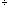Quandaries and Queries

Hi,

I am just curious whether Bedmas would be used in the following question as it is not in the typical Bedmas format.

Multiply     12 x 24
Divide by    2
Subtract     7

Would we go about doing it in the sequence it is given or in Bedmas ? I would appreciate your help in solving this problem.

Thanking you,
Gina

Hi Gina,

I don't think that bedmas comes into play here. Bedmas applies when you have an expression using mathematical notation. This problem has a multiplication followed by some further instructions in English. In this case I would follow the instructions in the order given.

Instruction Result
Multiply 1224 288
Divide by 2 157
Subtract 7 150

If the problem were written

1224 + 262 - 7

I would use bedmas and get

288 + 13 - 7 = 294

Penny

Go to Math Central